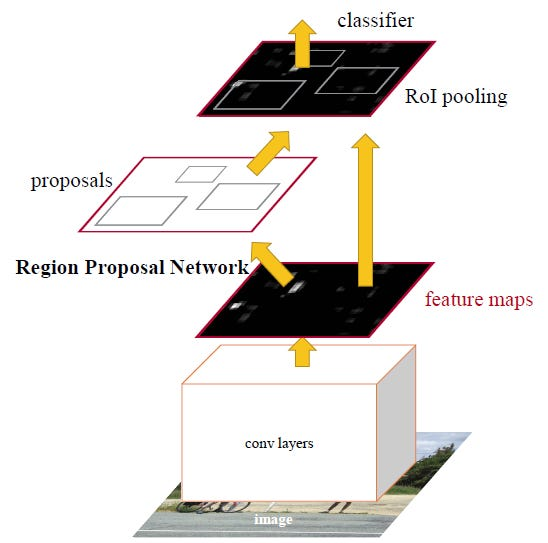Review: Faster R-CNN (Object Detection)

In this story, Faster R-CNN [1–2] is reviewed. In the previous Fast R-CNN  and R-CNN , region proposals are generated by selective search (SS)  rather than using convolutional neural network (CNN).

In Faster R-CNN [1–2], both region proposal generation and objection detection tasks are all done by the same conv networks. With such design, object detection is much faster.

To know deep learning object detection well, as a series of objection detection approaches, if there is enough time, it is better to read R-CNN, Fast R-CNN and Faster R-CNN in order, to know the evolution of objection detection, especially why region proposal network (RPN) is existed in this approach. I suggest to read my reviews about them if interested.

As Faster R-CNN is a state-of-the-art approach, it is published as 2015 NIPS paper and 2017 TPAMI paper with more than 4000 and 800 citations respectively when I was writing this story. (SH Tsang @ Medium)

What are covered

1. Region Proposal Network (RPN)
2. Detection Network
3. 4-Step Alternating Training
4. Ablation Study
5. Detection Results

1. Region Proposal Network (RPN)

In brief, R-CNN  and Fast R-CNN  first generate region proposals by selective search (SS) , then a CNN-based network is used to classify the object class and detect the bounding box. (The main difference is that R-CNN input the region proposals at pixel level into CNN for detection while Fast R-CNN input the region proposals at feature map level into CNN.) The region proposal approach/network (i.e. SS) and the detection network are decoupled.

Decoupling is not a good idea. Say for example, when SS has false negative, this error will hurt the detection network directly. It is better to couple them together such that they are correlated to each other, and update together by backpropagation.

In Faster R-CNN [1–2], RPN using SS  is replaced by RPN using CNN. And this CNN is shared with detection network. This CNN can be ZFNet or VGGNet in the paper. Thus, the overall network is as below:

1. First, the picture goes through conv layers and feature maps are extracted.
2. Then a sliding window is used in RPN for each location over the feature map.
3. For each location, k (k=9) anchor boxes are used (3 scales of 128, 256 and 512, and 3 aspect ratios of 1:1, 1:2, 2:1) for generating region proposals.
4. A cls layer outputs 2k scores whether there is object or not for k boxes.
5. A reg layer outputs 4k for the coordinates (box center coordinates, width and height) of k boxes.
6. With a size of W×H feature map, there are WHk anchors in total.

The average proposal size for 3 scales of 128, 256 and 512, and 3 aspect ratios of 1:1, 1:2, 2:1 are:

The loss function is:

The first term is the classification loss over 2 classes (There is object or not). The second term is the regression loss of bounding boxes only when there is object (i.e. p_i* =1).

Thus, RPN network is to pre-check which location contains object. And the corresponding locations and bounding boxes will pass to detection network for detecting the object class and returning the bounding box of that object.

As regions can be highly overlapped with each other, non-maximum suppression (NMS) is used to reduce the number of proposals from about 6000 to N (N=300).

2. Detection Network

Except the RPN, the remaining part is similar to the Fast R-CNN. ROI pooling is performed first. And then the pooled area goes through CNN and two FC branches for class softmax and bounding box regressor. (If interested, please read my review about Fast R-CNN.)

3. 4-Step Alternating Training

Since the conv layers are shared to extract the feature maps with different outputs at the end, thus, training procedure is quite different:

1. Train (fine-tune) RPN with imagenet pre-trained model.
2. Train (fine-tune) a separate detection network with imagenet pre-trained model. (Conv layers not yet shared)
3. Use the detector network to initialize PRN training, fix the shared conv layers, only fine-tune unique layers of RPN.
4. Keep the conv layers fixed, fine-tune the unique layers of detector network.

4. Ablation Study

4.1. Region Proposal

As mentioned, with unshared conv layer (Only first 2 steps in alternating training), 58.7% mAP is obtained. With shared conv layers, 59.9% mAP is obtained. And it is better than prior arts SS and EB.

4.2 Scales and Ratios

With 3 scales and 3 ratios, 69.9% mAP is obtained which is only little improvement over that of 3 scales and 1 ratio. But still 3 scales and 3 ratios are used.

4.3 λ in Loss Function

λ = 10 achieves the best result.

5. Detection Results

5.1 PASCAL VOC 2007

With training data using COCO, VOC 2007 (trainval) and VOC 2012 (trainval) dataset, 78.8% mAP is obtained.

5.2 PASCAL VOC 2012

With training data using COCO, VOC 2007 (trainval+test) and VOC 2012 (trainval) dataset, 75.9% mAP is obtained.

5.3 MS COCO

42.1% mAP is obtained with IoU @ 0.5 using COCO train set for training.
21.5% mAP is obtained with IoU from 0.5 to 0.95 with step size of 0.05.